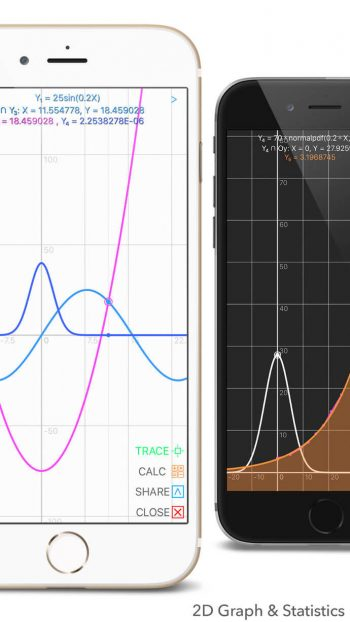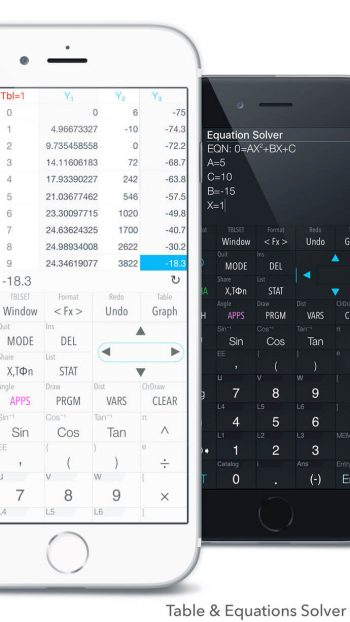# Graphing Calculator Plus

Graphing Calculator has a formidable reputation of being the top alternative to heavy complex hardware calculators. It is suitable for all types of users, whether they are looking to carry out basic or advanced mathematics; Graphing Calculator has it all. It is equipped with a powerful MATH CORE advocated by MATH DISPLAY support, which increases its functionality by leaps and bounds, making it an ideal choice for engineers, math students etc. It comes with an in depth User Manual and Tutorial to answer any questions you have, regarding the app and to help you further understand the world of advanced mathematics.

• Developer
Incpt.Mobis
PRICE: \$4.99## Product Description

Graphing Calculator has a formidable reputation of being the top alternative to heavy complex hardware calculators. It is suitable for all types of users, whether they are looking to carry out basic or advanced mathematics; Graphing Calculator has it all. It is equipped with a powerful MATH CORE advocated by MATH DISPLAY support, which increases its functionality by leaps and bounds, making it an ideal choice for engineers, math students etc. It comes with an in depth User Manual and Tutorial to answer any questions you have, regarding the app and to help you further understand the world of advanced mathematics.

◉ BUILT-IN DOCUMENTS:
▸ Basic General Calculation
▸ X & Y Calculation & Intersection of Functions
▸ Calculate Local Maxima, Minima…
▸ Statistics & Regression
▸ Solve Equations & Polynomial
▸ Calculate Integral Functions

◉ CALCULATOR INCLUDED:
▸ Universal Calculator App
▸ Mathematical Display
▸ Complex Number Calculation
▸ Equations Solver
▸ Edit & Export Expression/Result
▸ History Tape View
▸ Basic Calculations (+, -, x, ÷, ^, √,…)
▸ Y-Var & Customize Functions (F1, F2, F3)
▸ Unit Converter (50 Categories, 1000+ Units)
▸ Over 40 Common Constants
▸ Fraction and Degree/Angle Calculation
▸ Numeric Integral & Derivative Calculation
▸ Dual Themes(Skin)

◉ 2D GRAPH:
▸ Simultaneous Graph
▸ Smooth Zoom & Transformation
▸ Support Parametric, Polar & Sequences
▸ Intersections, Min, Max & Special Points TRACE

◉ STATISTIC,REGRESSION & TEST:
▸ Create/Edit Lists
▸ 1-Var, 2-Var Statistic
▸ Logarithm, Exponential, Power,… Regression
▸ PropZ,T, SampZ,T, Chi, Interval… Test

◉ MATRIX:
▸ Create/Edit Matrices
▸ Basic Matrix Functions (Det,Dim,Fill,…)
▸ Advanced Matrix Functions (Inv, Ref, RRef,…)

◉ PROGRAMING:
▸ Control Commands
▸ I/O Commands
▸ Example Source Codes Included

◉ NUMBER BASE-N MODE:
▸ 15 Different Bases
▸ Support Up to 64 Bits Integer
▸ Binary Operation (NOT, OR, XOR, 2’S,…)